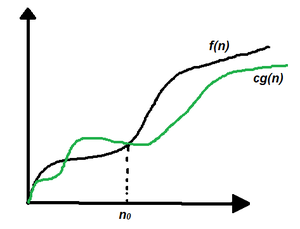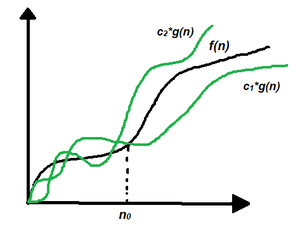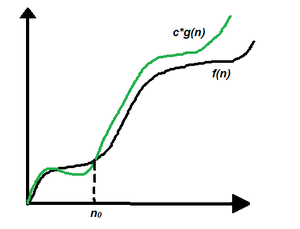Related Articles

# Asymptotic Notations and how to calculate them

• Difficulty Level : Expert
• Last Updated : 14 Jul, 2021

In mathematics, asymptotic analysis, also known as asymptotics, is a method of describing the limiting behavior of a function. In computing, asymptotic analysis of an algorithm refers to defining the mathematical boundation of its run-time performance based on the input size. For example, the running time of one operation is computed as f(n), and maybe for another operation, it is computed as g(n2). This means the first operation running time will increase linearly with the increase in n and the running time of the second operation will increase exponentially when n increases. Similarly, the running time of both operations will be nearly the same if n is small in value.

Attention reader! Don’t stop learning now. Get hold of all the important DSA concepts with the DSA Self Paced Course at a student-friendly price and become industry ready.  To complete your preparation from learning a language to DS Algo and many more,  please refer Complete Interview Preparation Course.

In case you wish to attend live classes with experts, please refer DSA Live Classes for Working Professionals and Competitive Programming Live for Students.

1. Best Case (Omega Notation (Ω))
2. Average Case (Theta Notation (Ω))
3. Worst Case (O Notation(O))

All of these notations are discussed below in details:

Omega (Ω) Notation:

Omega (Ω) notation specifies the asymptotic lower bound for a function f(n). For a given function g(n), Ω(g(n)) is denoted by:

Ω (g(n)) = {f(n): there exist positive constants c and n0 such that 0 ≤ c*g(n) ≤ f(n) for all n ≥ n0}.

This means that, f(n) = Ω(g(n)), If there are positive constants n0 and c such that, to the right of n0 the f(n) always lies on or above c*g(n).Graphical representation

Follow the steps below to calculate Ω for a program:

1. Break the program into smaller segments.
2. Find the number of operations performed for each segment(in terms of the input size) assuming the given input is such that the program takes the least amount of time.
3. Add up all the operations and simplify it, let’s say it is f(n).
4. Remove all the constants and choose the term having the least order or any other function which is always less than f(n) when n tends to infinity, let say it is g(n) then, Omega (Ω) of f(n) is Ω(g(n)).

Omega notation doesn’t really help to analyze an algorithm because it is bogus to evaluate an algorithm for the best cases of inputs.

Theta (Θ) Notation:

Big-Theta(Θ) notation specifies a bound for a function f(n). For a given function g(n), Θ(g(n)) is denoted by:

Θ (g(n)) = {f(n): there exist positive constants c1, c2 and n0 such that 0 ≤ c1*g(n) ≤ f(n) ≤ c2*g(n) for all n ≥ n0}.

This means that, f(n) = Θ(g(n)), If there are positive constants n0 and c such that, to the right of n0 the f(n) always lies on or above c1*g(n) and below c2*g(n).Graphical representation

Follow the steps below to calculate Θ for a program:

1. Break the program into smaller segments.
2. Find all types of inputs and calculate the number of operations they take to be executed. Make sure that the input cases are equally distributed.
3. Find the sum of all the calculated values and divide the sum by the total number of inputs let say the function of n obtained is g(n) after removing all the constants, then in Θ notation, it’s represented as Θ(g(n)).

Example: In a linear search problem, let’s assume that all the cases are uniformly distributed (including the case when the key is absent in the array). So, sum all the cases when the key is present at positions 1, 2, 3, ……, n and not present, and divide the sum by n + 1.

Average case time complexity =⇒⇒⇒Since all the types of inputs are considered while calculating the average time complexity, it is one of the best analysis methods for an algorithm.

Big – O Notation:

Big – O (O) notation specifies the asymptotic upper bound for a function f(n). For a given function g(n), O(g(n)) is denoted by:

Ω (g(n)) = {f(n): there exist positive constants c and n0 such that f(n) ≤ c*g(n) for all n ≥ n0}.

This means that, f(n) = Ω(g(n)), If there are positive constants n0 and c such that, to the right of n0 the f(n) always lies on or below c*g(n).Graphical representation

Follow the steps below to calculate O for a program:

1. Break the program into smaller segments.
2. Find the number of operations performed for each segment (in terms of the input size) assuming the given input is such that the program takes the maximum time i.e the worst-case scenario.
3. Add up all the operations and simplify it, let’s say it is f(n).
4. Remove all the constants and choose the term having the highest order because for n tends to infinity the constants and the lower order terms in f(n) will be insignificant, let say the function is g(n) then, big-O notation is O(g(n)).

It is the most widely used notation as it is easier to calculate since there is no need to check for every type of input as it was in the case of theta notation, also since the worst case of input is taken into account it pretty much gives the upper bound of the time the program will take to execute.

My Personal Notes arrow_drop_up